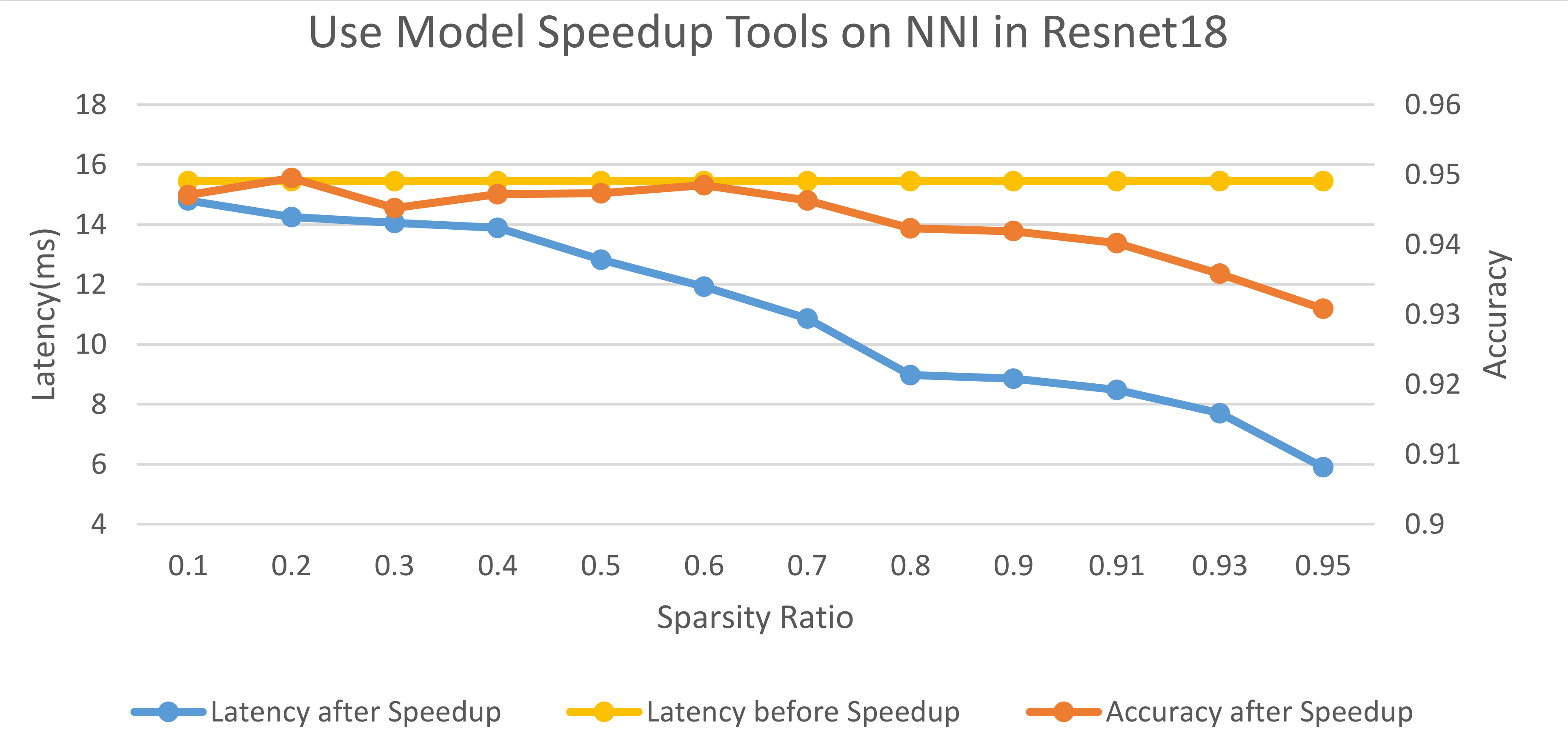## Introduction¶

Pruning algorithms usually use weight masks to simulate the real pruning. Masks can be used to check model performance of a specific pruning (or sparsity), but there is no real speedup. Since model speedup is the ultimate goal of model pruning, we try to provide a tool to users to convert a model to a smaller one based on user provided masks (the masks come from the pruning algorithms).

There are two types of pruning. One is fine-grained pruning, it does not change the shape of weights, and input/output tensors. Sparse kernel is required to speedup a fine-grained pruned layer. The other is coarse-grained pruning (e.g., channels), shape of weights and input/output tensors usually change due to such pruning. To speedup this kind of pruning, there is no need to use sparse kernel, just replace the pruned layer with smaller one. Since the support of sparse kernels in community is limited, we only support the speedup of coarse-grained pruning and leave the support of fine-grained pruning in future.

## Design and Implementation¶

To speedup a model, the pruned layers should be replaced, either replaced with smaller layer for coarse-grained mask, or replaced with sparse kernel for fine-grained mask. Coarse-grained mask usually changes the shape of weights or input/output tensors, thus, we should do shape inference to check are there other unpruned layers should be replaced as well due to shape change. Therefore, in our design, there are two main steps: first, do shape inference to find out all the modules that should be replaced; second, replace the modules.

The first step requires topology (i.e., connections) of the model, we use a tracer based on `torch.fx` to obtain the model graph for PyTorch. The new shape of module is auto-inference by NNI, the unchanged parts of outputs during forward and inputs during backward are prepared for reduct. For each type of module, we should prepare a function for module replacement. The module replacement function returns a newly created module which is smaller.

## Usage¶

Generate a mask for the model at first. We usually use a NNI pruner to generate the masks then use `ModelSpeedup` to compact the model. But in fact `ModelSpeedup` is a relatively independent tool, so you can use it independently.

```import torch
from nni_assets.compression.mnist_model import TorchModel, device

model = TorchModel().to(device)
# mask the first three output channels in conv1
```

Show the original model structure.

```print(model)
```
```TorchModel(
(conv1): Conv2d(1, 6, kernel_size=(5, 5), stride=(1, 1))
(conv2): Conv2d(6, 16, kernel_size=(5, 5), stride=(1, 1))
(fc1): Linear(in_features=256, out_features=120, bias=True)
(fc2): Linear(in_features=120, out_features=84, bias=True)
(fc3): Linear(in_features=84, out_features=10, bias=True)
(relu1): ReLU()
(relu2): ReLU()
(relu3): ReLU()
(relu4): ReLU()
(pool1): MaxPool2d(kernel_size=(2, 2), stride=(2, 2), padding=0, dilation=1, ceil_mode=False)
(pool2): MaxPool2d(kernel_size=(2, 2), stride=(2, 2), padding=0, dilation=1, ceil_mode=False)
)
```

Roughly test the original model inference speed.

```import time
start = time.time()
model(torch.rand(128, 1, 28, 28).to(device))
print('Original Model - Elapsed Time : ', time.time() - start)
```
```Original Model - Elapsed Time :  2.3036391735076904
```

Speedup the model and show the model structure after speedup.

```from nni.compression.speedup import ModelSpeedup
ModelSpeedup(model, torch.rand(10, 1, 28, 28).to(device), masks).speedup_model()
print(model)
```
```TorchModel(
(conv1): Conv2d(1, 3, kernel_size=(5, 5), stride=(1, 1))
(conv2): Conv2d(3, 16, kernel_size=(5, 5), stride=(1, 1))
(fc1): Linear(in_features=256, out_features=120, bias=True)
(fc2): Linear(in_features=120, out_features=84, bias=True)
(fc3): Linear(in_features=84, out_features=10, bias=True)
(relu1): ReLU()
(relu2): ReLU()
(relu3): ReLU()
(relu4): ReLU()
(pool1): MaxPool2d(kernel_size=(2, 2), stride=(2, 2), padding=0, dilation=1, ceil_mode=False)
(pool2): MaxPool2d(kernel_size=(2, 2), stride=(2, 2), padding=0, dilation=1, ceil_mode=False)
)
```

Roughly test the model after speedup inference speed.

```start = time.time()
model(torch.rand(128, 1, 28, 28).to(device))
print('Speedup Model - Elapsed Time : ', time.time() - start)
```
```Speedup Model - Elapsed Time :  0.09416508674621582
```

For combining usage of `Pruner` masks generation with `ModelSpeedup`, please refer to Pruning Quick Start.

NOTE: The current implementation supports PyTorch 1.3.1 or newer.

## Limitations¶

For PyTorch we can only replace modules, if functions in `forward` should be replaced, our current implementation does not work. One workaround is make the function a PyTorch module.

If you want to speedup your own model which cannot supported by the current implementation, you need implement the replace function for module replacement, welcome to contribute.

## Speedup Results of Examples¶

These result are tested on the legacy pruning framework, new results will coming soon.

### slim pruner example¶

on one V100 GPU, input tensor: `torch.randn(64, 3, 32, 32)`

Times

Speedup Latency

1

0.01197

0.005107

2

0.02019

0.008769

4

0.02733

0.014809

8

0.04310

0.027441

16

0.07731

0.05008

32

0.14464

0.10027

### fpgm pruner example¶

on cpu, input tensor: `torch.randn(64, 1, 28, 28)`, too large variance

Times

Speedup Latency

1

0.01383

0.01839

2

0.01167

0.003558

4

0.01636

0.01088

40

0.14412

0.08268

40

1.29385

0.14408

40

0.41035

0.46162

400

6.29020

5.82143

### l1filter pruner example¶

on one V100 GPU, input tensor: `torch.randn(64, 3, 32, 32)`

Times

Speedup Latency

1

0.01026

0.003677

2

0.01657

0.008161

4

0.02458

0.020018

8

0.03498

0.025504

16

0.06757

0.047523

32

0.10487

0.086442

### APoZ pruner example¶

on one V100 GPU, input tensor: `torch.randn(64, 3, 32, 32)`

Times

Speedup Latency

1

0.01389

0.004208

2

0.01628

0.008310

4

0.02521

0.014008

8

0.03386

0.023923

16

0.06042

0.046183

32

0.12421

0.087113

### SimulatedAnnealing pruner example¶

In this experiment, we use SimulatedAnnealing pruner to prune the resnet18 on the cifar10 dataset. We measure the latencies and accuracies of the pruned model under different sparsity ratios, as shown in the following figure. The latency is measured on one V100 GPU and the input tensor is `torch.randn(128, 3, 32, 32)`.Total running time of the script: ( 0 minutes 10.330 seconds)

Gallery generated by Sphinx-Gallery